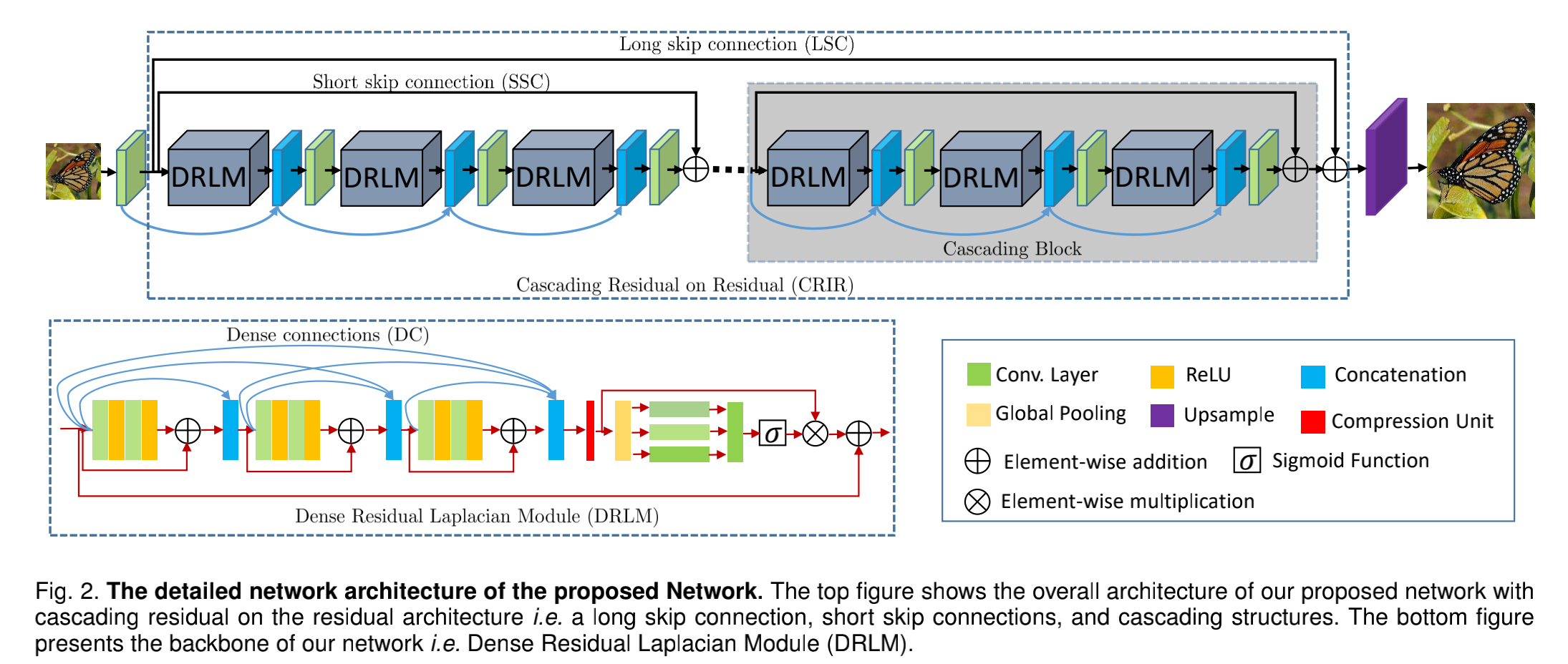# [Paper Note] Densely Residual Laplacian Super-Resolution

### Intro

• 我们提出了密集连接的残差块和拉普拉斯注意网络，以实现精确的图像超分辨率。 我们的网络通过多快捷连接和多级表示实现了更好的性能
• 我们的新颖设计在残差架构上采用级联残差，可以帮助训练深层网络。 多样化的连接类型和DRLN中残差上的残差的级联有助于绕过足够的低频信息以学习更准确的表示
• 提出了拉普拉斯注意力机制，这个机制主要有两个目的：1）学习多个子带频率的特征，2）自适应不同尺度特征和模型特征依赖性。 拉普拉斯注意力进一步提高了我们网络的特征捕获能力
• 通过大量实验，我们证明DRLN是有效的
有利于实现更好的性能

### Model$f_{0}=H_{f}(x) \tag{1}$
$H_{f}(\cdot)$是应用于低分辨率图像的卷积算子,接下来，将$f_0$传递给残差模块上的级联残差，称为$H_{crir}$
$f_{r}=H_{c r i r}\left(f_{0}\right) \tag{2}$

$f_{u}=H_{u}\left(f_{r}\right) \tag{3}$
$H_u(\cdot)$$f_u$ 各自表示上采样操作和上采样后的特征。虽然$H_u(\cdot）$有多种选择，例如反卷积层，或最近邻上采样卷积; 我们选择ESPCN 跟随，的脚步。 接下来，$f_u$特征传到重建组件，该组件由一个卷积层组成，以预测超分辨RGB颜色通道作为输出，表示为
$\hat{y}=H_{r}\left(f_{u}\right) \tag{4}$

$L(\mathcal{W})=\frac{1}{N} \sum_{i=1}^{N}\left\|\operatorname{DRLN}\left(x_{i}\right)-y_{i}\right\|_{1} \tag{5}$
$DRLN(\cdot)$是网络，$W$是参数集合

#### 残差上的级联残差

$f_{n, m}=f\left(\left[Z^{u-0 ; m}, Z^{u-1 ; m}, B^{u ; m}\left(w^{u, 1 ; m}\right), b^{u, 1 ; m}\right)\right. \tag{6}$

$f_{g}=f_{0}+H_{c r i r}\left(\mathcal{W}_{w, b}\right) \tag{7}$

#### 稠密残差 拉普拉斯模块

$R_{i}\left(w_{i}, b_{i}\right)=\tau\left(f(\tau)\left(f\left(w_{i, 1}, b_{i, 1}\right) ; b_{i, 2}\right)+Z_{i-1}\right) \tag{8}$

$\begin{array}{l}{R_{c}=\left[R_{i-1}\left(w_{i-1}, b_{i-1}\right) ; R_{i-2}\left(w_{i-2}, b_{i-2}\right)\right]} \\ {f_{R}=R_{i}\left(w_{i}, b_{i}\right)=\tau\left(f\left(f\left(f_{c}\right)\right)+R_{c}\right)}\end{array} \tag{9}$

$g_{d}=\frac{1}{h \times w} \sum_{i=1}^{h} \sum_{i=1}^{w} f_{c}(i, j) \tag{10}$

\begin{aligned} r_{3} &=\tau\left(D_{f_{3}}\left(g_{d}\right)\right) \\ r_{5} &=\tau\left(D_{f_{5}}\left(g_{d}\right)\right) \\ r_{7} &=\tau\left(D_{f_{7}}\left(g_{d}\right)\right) \end{aligned} \tag{11}

$g_{p}=\left[r_{3} ; r_{5} ; r_{7}\right] \tag{12}$

$L_{p}=\sigma\left(U_{f}\left(g_{p}\right)\right) \tag{13}$

$\hat{f}_{c}=L_{p} \times f_{c} \tag{14}$